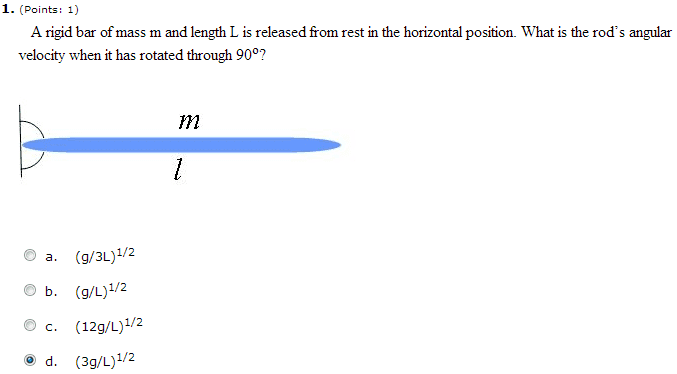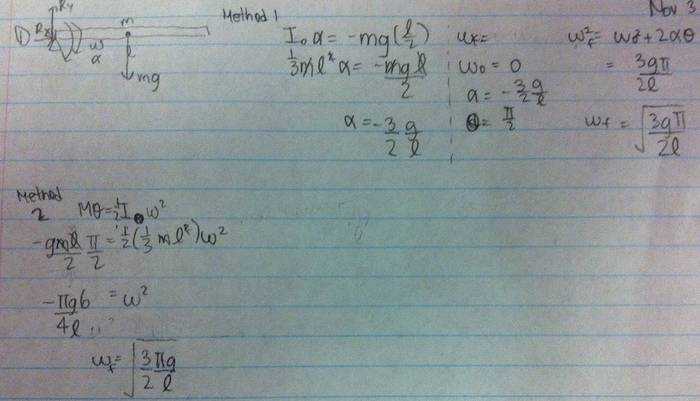# Finding angular velocity using work energy theorem

## Homework Statement## Homework Equations

Work done by rotation = M*theta = 0.5(Inertia about pin) *ω2

## The Attempt at a Solution

I tried two different methods and both came out to be the same answer. However they did not correspond to any of the multiple choice answers... What am I doing wrong?Thanks!

Method 1: The angular acceleration varies with $\cos(\theta)$. So using constant acceleration formulas doesn't work. You simply can't use that method.

Method 2: The potential energy is still $U=mgh$, not $U=M\theta$.

Method 1: The angular acceleration varies with $\cos(\theta)$. So using constant acceleration formulas doesn't work. You simply can't use that method.

Method 2: The potential energy is still $U=mgh$, not $U=M\theta$.

Thanks, but why does Mtheta not work?

I'm not even sure what M is.

I'm not even sure what M is.

It's still wrong. It should be $\frac{1}{2}M\omega$. But that by itself contains no information about the fact it's in gravity.